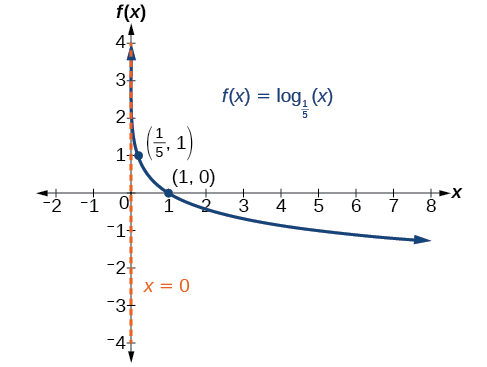# 6.4 Graphs of logarithmic functions  (Page 3/8)

 Page 3 / 8

Given a logarithmic function with the form $\text{\hspace{0.17em}}f\left(x\right)={\mathrm{log}}_{b}\left(x\right),$ graph the function.

1. Draw and label the vertical asymptote, $\text{\hspace{0.17em}}x=0.$
2. Plot the x- intercept, $\text{\hspace{0.17em}}\left(1,0\right).$
3. Plot the key point $\text{\hspace{0.17em}}\left(b,1\right).$
4. Draw a smooth curve through the points.
5. State the domain, $\text{\hspace{0.17em}}\left(0,\infty \right),$ the range, $\text{\hspace{0.17em}}\left(-\infty ,\infty \right),$ and the vertical asymptote, $\text{\hspace{0.17em}}x=0.$

## Graphing a logarithmic function with the form f ( x ) = log b ( x ).

Graph $\text{\hspace{0.17em}}f\left(x\right)={\mathrm{log}}_{5}\left(x\right).\text{\hspace{0.17em}}$ State the domain, range, and asymptote.

Before graphing, identify the behavior and key points for the graph.

• Since $\text{\hspace{0.17em}}b=5\text{\hspace{0.17em}}$ is greater than one, we know the function is increasing. The left tail of the graph will approach the vertical asymptote $\text{\hspace{0.17em}}x=0,$ and the right tail will increase slowly without bound.
• The x -intercept is $\text{\hspace{0.17em}}\left(1,0\right).$
• The key point $\text{\hspace{0.17em}}\left(5,1\right)\text{\hspace{0.17em}}$ is on the graph.
• We draw and label the asymptote, plot and label the points, and draw a smooth curve through the points (see [link] ).

The domain is $\text{\hspace{0.17em}}\left(0,\infty \right),$ the range is $\text{\hspace{0.17em}}\left(-\infty ,\infty \right),$ and the vertical asymptote is $\text{\hspace{0.17em}}x=0.$

Graph $\text{\hspace{0.17em}}f\left(x\right)={\mathrm{log}}_{\frac{1}{5}}\left(x\right).\text{\hspace{0.17em}}$ State the domain, range, and asymptote.The domain is $\text{\hspace{0.17em}}\left(0,\infty \right),$ the range is $\text{\hspace{0.17em}}\left(-\infty ,\infty \right),$ and the vertical asymptote is $\text{\hspace{0.17em}}x=0.$

## Graphing transformations of logarithmic functions

As we mentioned in the beginning of the section, transformations of logarithmic graphs behave similarly to those of other parent functions. We can shift, stretch, compress, and reflect the parent function $\text{\hspace{0.17em}}y={\mathrm{log}}_{b}\left(x\right)\text{\hspace{0.17em}}$ without loss of shape.

## Graphing a horizontal shift of f ( x ) = log b ( x )

When a constant $\text{\hspace{0.17em}}c\text{\hspace{0.17em}}$ is added to the input of the parent function $\text{\hspace{0.17em}}f\left(x\right)=lo{g}_{b}\left(x\right),$ the result is a horizontal shift     $\text{\hspace{0.17em}}c\text{\hspace{0.17em}}$ units in the opposite direction of the sign on $\text{\hspace{0.17em}}c.\text{\hspace{0.17em}}$ To visualize horizontal shifts, we can observe the general graph of the parent function $f\left(x\right)={\mathrm{log}}_{b}\left(x\right)\text{\hspace{0.17em}}$ and for $\text{\hspace{0.17em}}c>0\text{\hspace{0.17em}}$ alongside the shift left, $\text{\hspace{0.17em}}g\left(x\right)={\mathrm{log}}_{b}\left(x+c\right),$ and the shift right, $\text{\hspace{0.17em}}h\left(x\right)={\mathrm{log}}_{b}\left(x-c\right).$ See [link] .

## Horizontal shifts of the parent function y = log b ( x )

For any constant $\text{\hspace{0.17em}}c,$ the function $\text{\hspace{0.17em}}f\left(x\right)={\mathrm{log}}_{b}\left(x+c\right)$

• shifts the parent function $\text{\hspace{0.17em}}y={\mathrm{log}}_{b}\left(x\right)\text{\hspace{0.17em}}$ left $\text{\hspace{0.17em}}c\text{\hspace{0.17em}}$ units if $\text{\hspace{0.17em}}c>0.$
• shifts the parent function $\text{\hspace{0.17em}}y={\mathrm{log}}_{b}\left(x\right)\text{\hspace{0.17em}}$ right $\text{\hspace{0.17em}}c\text{\hspace{0.17em}}$ units if $\text{\hspace{0.17em}}c<0.$
• has the vertical asymptote $\text{\hspace{0.17em}}x=-c.$
• has domain $\text{\hspace{0.17em}}\left(-c,\infty \right).$
• has range $\text{\hspace{0.17em}}\left(-\infty ,\infty \right).$

Given a logarithmic function with the form $\text{\hspace{0.17em}}f\left(x\right)={\mathrm{log}}_{b}\left(x+c\right),$ graph the translation.

1. Identify the horizontal shift:
1. If $\text{\hspace{0.17em}}c>0,$ shift the graph of $\text{\hspace{0.17em}}f\left(x\right)={\mathrm{log}}_{b}\left(x\right)\text{\hspace{0.17em}}$ left $\text{\hspace{0.17em}}c\text{\hspace{0.17em}}$ units.
2. If $\text{\hspace{0.17em}}c<0,$ shift the graph of $\text{\hspace{0.17em}}f\left(x\right)={\mathrm{log}}_{b}\left(x\right)\text{\hspace{0.17em}}$ right $\text{\hspace{0.17em}}c\text{\hspace{0.17em}}$ units.
2. Draw the vertical asymptote $\text{\hspace{0.17em}}x=-c.$
3. Identify three key points from the parent function. Find new coordinates for the shifted functions by subtracting $\text{\hspace{0.17em}}c\text{\hspace{0.17em}}$ from the $\text{\hspace{0.17em}}x\text{\hspace{0.17em}}$ coordinate.
4. Label the three points.
5. The Domain is $\text{\hspace{0.17em}}\left(-c,\infty \right),$ the range is $\text{\hspace{0.17em}}\left(-\infty ,\infty \right),$ and the vertical asymptote is $\text{\hspace{0.17em}}x=-c.$

## Graphing a horizontal shift of the parent function y = log b ( x )

Sketch the horizontal shift $\text{\hspace{0.17em}}f\left(x\right)={\mathrm{log}}_{3}\left(x-2\right)\text{\hspace{0.17em}}$ alongside its parent function. Include the key points and asymptotes on the graph. State the domain, range, and asymptote.

Since the function is $\text{\hspace{0.17em}}f\left(x\right)={\mathrm{log}}_{3}\left(x-2\right),$ we notice $\text{\hspace{0.17em}}x+\left(-2\right)=x–2.$

Thus $\text{\hspace{0.17em}}c=-2,$ so $\text{\hspace{0.17em}}c<0.\text{\hspace{0.17em}}$ This means we will shift the function $\text{\hspace{0.17em}}f\left(x\right)={\mathrm{log}}_{3}\left(x\right)\text{\hspace{0.17em}}$ right 2 units.

The vertical asymptote is $\text{\hspace{0.17em}}x=-\left(-2\right)\text{\hspace{0.17em}}$ or $\text{\hspace{0.17em}}x=2.$

Consider the three key points from the parent function, $\text{\hspace{0.17em}}\left(\frac{1}{3},-1\right),$ $\left(1,0\right),$ and $\text{\hspace{0.17em}}\left(3,1\right).$

The new coordinates are found by adding 2 to the $\text{\hspace{0.17em}}x\text{\hspace{0.17em}}$ coordinates.

Label the points $\text{\hspace{0.17em}}\left(\frac{7}{3},-1\right),$ $\left(3,0\right),$ and $\text{\hspace{0.17em}}\left(5,1\right).$

The domain is $\text{\hspace{0.17em}}\left(2,\infty \right),$ the range is $\text{\hspace{0.17em}}\left(-\infty ,\infty \right),$ and the vertical asymptote is $\text{\hspace{0.17em}}x=2.$

what are odd numbers
numbers that leave a remainder when divided by 2
Thorben
1,3,5,7,... 99,...867
Thorben
7%2=1, 679%2=1, 866245%2=1
Thorben
the third and the seventh terms of a G.P are 81 and 16, find the first and fifth terms.
if a=3, b =4 and c=5 find the six trigonometric value sin
pls how do I factorize x⁴+x³-7x²-x+6=0
in a function the input value is called
how do I test for values on the number line
if a=4 b=4 then a+b=
a+b+2ab
Kin
commulative principle
a+b= 4+4=8
Mimi
If a=4 and b=4 then we add the value of a and b i.e a+b=4+4=8.
Tariq
what are examples of natural number
an equation for the line that goes through the point (-1,12) and has a slope of 2,3
3y=-9x+25
Ishaq
show that the set of natural numberdoes not from agroup with addition or multiplication butit forms aseni group with respect toaaddition as well as multiplication
x^20+x^15+x^10+x^5/x^2+1
evaluate each algebraic expression. 2x+×_2 if ×=5
if the ratio of the root of ax+bx+c =0, show that (m+1)^2 ac =b^2m
By the definition, is such that 0!=1.why?
(1+cosA+IsinA)(1+cosB+isinB)/(cos@+isin@)(cos$+isin$)
hatdog
Mark
jaks
Ryan

#### Get Jobilize Job Search Mobile App in your pocket Now!ByByBy OpenStaxBy OpenStaxBy OpenStaxBy Sebastian Sieczko...By Brianna BeckBy Dakota BocanBy OpenStaxByBy OpenStaxBy Madison Christian